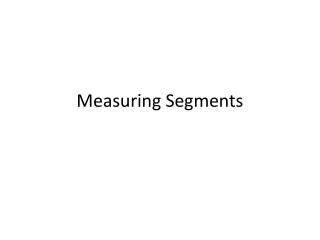DownloadDownload PresentationMeasuring Segments

# Measuring Segments

Télécharger la présentation## Measuring Segments

- - - - - - - - - - - - - - - - - - - - - - - - - - - E N D - - - - - - - - - - - - - - - - - - - - - - - - - - -
##### Presentation Transcript

1. Measuring Segments

2. Length on a Number Line • The distance between any two points is the absolute value of the ______________ of the coordinates. • Ex. Find each length. 1. DC 2. EF

3. Congruent Segments • Congruent segments are segments that have the same ___________. • The congruence symbol is _______. • We show two segments are congruent by drawing tick marks.

4. Segment Addition Postulate • If Bis between A and C, then AB + BC = AC • Ex. 1 B is between A and C; AC = 14, BC = 11.4. Find AB. (hint: draw a picture!)

5. Ex. 2 S is between R and T. Find RT.

6. Midpoints • The midpoint of a segment is the point that ______________, or divides, the segment into two congruent segments. • Use a diagram to show the midpoint.

7. Ex. 1 The map shows the route for a race. You are 365m from drink station R and 2km from drink station S. The first aid station is at the midpoint of the two drink stations. How far are you from the first aid station?

8. Ex. 2